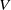Hostname: page-component-5db6c4db9b-bhjbq Total loading time: 0 Render date: 2023-03-25T04:33:52.584Z Has data issue: true Feature Flags: { "useRatesEcommerce": false } hasContentIssue true

# Dimensions of random affine code tree fractals

Published online by Cambridge University Press:  30 January 2013

## Abstract

We study the dimension of code tree fractals, a class of fractals generated by a set of iterated function systems. We first consider deterministic affine code tree fractals, extending to the code tree fractal setting the classical result of Falconer and Solomyak on the Hausdorff dimension of self-affine fractals generated by a single iterated function system. We then calculate the almost sure Hausdorff, packing and box counting dimensions of a general class of random affine planar code tree fractals. The set of probability measures describing the randomness includes natural measures in random$V$-variable and homogeneous Markov constructions.

Type
Research Article
Information
Ergodic Theory and Dynamical Systems , June 2014 , pp. 854 - 875

## Access options

Get access to the full version of this content by using one of the access options below. (Log in options will check for institutional or personal access. Content may require purchase if you do not have access.)

## References

Barnsley, M., Hutchinson, J. E. and Stenflo, Ö.. A fractal valued random iteration algorithm and fractal hierarchy. Fractals 13 (2005), 111146.CrossRefGoogle Scholar
Barnsley, M., Hutchinson, J. E. and Stenflo, Ö.. V-variable fractals: fractals with partial self similarity. Adv. Math. 218 (2008), 20512088.CrossRefGoogle Scholar
Barnsley, M., Hutchinson, J. E. and Stenflo, Ö.. V-variable fractals: dimension results. Forum Math. 24 (2012), 445470.CrossRefGoogle Scholar
Bougerol, P. and Lacroix, J.. Products of Random Matrices with Applications to Schrödinger Operators. Birkhäuser, 1985.CrossRefGoogle Scholar
Durrett, R.. Probability: Theory and Examples. Wadsworth & Brooks/Cole, Pacific Grove, CA, 1991.Google Scholar
Edgar, G. A.. Fractal dimension of self-affine sets: some examples. Rend. Circ. Mat. Palermo (2) Suppl. 28 (1992), 341358.Google Scholar
Erdös, P.. On a family of symmetric Bernoulli convolutions. Amer. J. Math. 61 (1939), 974976.CrossRefGoogle Scholar
Falconer, K. J.. Random fractals. Math. Proc. Cambridge Philos. Soc. 100 (1986), 559582.CrossRefGoogle Scholar
Falconer, K. J.. The Hausdorff dimension of self-affine fractals. Math. Proc. Cambridge Philos. Soc. 103 (1988), 339350.CrossRefGoogle Scholar
Falconer, K. J.. Sub-self-similar sets. Trans. Amer. Math. Soc. 347 (1995), 31213129.CrossRefGoogle Scholar
Falconer, K. J.. Techniques in Fractal Geometry. John Wiley & Sons, 1997.Google Scholar
Falconer, K. J.. Fractal Geometry. John Wiley & Sons, 2006.Google Scholar
Falconer, K. J. and Miao, J.. Random subsets of self-affine fractals. Mathematika 56 (2010), 6176.CrossRefGoogle Scholar
Feng, D. J.. Lyapunov exponents for products of matrices and multifractal analysis, Part II: general matrices. Israel J. Math. 170 (2009), 355394.CrossRefGoogle Scholar
Gatzouras, D. and Lalley, S. P.. Statistically self-affine sets: Hausdorff and box dimensions. J. Theoret. Probab. 7 (1994), 437468.CrossRefGoogle Scholar
Hutchinson, J. E.. Fractals and self-similarity. Indiana Univ. Math. J. 30 (1981), 713747.CrossRefGoogle Scholar
Jordan, T., Pollicott, M. and Simon, K.. Hausdorff dimension for randomly perturbed self affine attractors. Comm. Math. Phys. 270 (2007), 519544.CrossRefGoogle Scholar
Käenmäki, A. and Vilppolainen, M.. Dimension and measures on sub-self-affine sets. Monatsh. Math. 161 (2010), 271293.CrossRefGoogle Scholar
Mauldin, R. D. and Urbański, M.. Graph Directed Markov Systems–Geometry and Dynamics of Limit Sets (Cambridge Tracts in Mathematics, 148). Cambridge University Press, Cambridge, 2003.CrossRefGoogle Scholar
Pesin, Y. and Weiss, H.. On the dimension of deterministic and random Cantor-like sets, symbolic dynamics, and the Eckmann–Ruelle conjecture. Comm. Math. Phys. 182 (1996), 105153.CrossRefGoogle Scholar
Przytycki, F. and Urbański, M.. On the Hausdorff dimension of some fractal sets. Studia Math. 93 (1989), 155186.CrossRefGoogle Scholar
Rajala, T. and Vilppolainen, M.. Weakly controlled Moran constructions and iterated function systems in metric spaces. Illinois J. Math. to appear.Google Scholar
Simon, K. and Solomyak, B.. On the dimension of self-similar sets. Fractals 10 (2002), 5965.CrossRefGoogle Scholar
Solomyak, B.. Measure and dimensions for some fractal families. Math. Proc. Cambridge Philos. Soc. 124 (1998), 531546.CrossRefGoogle Scholar